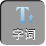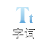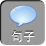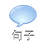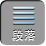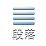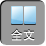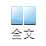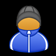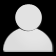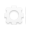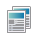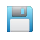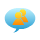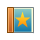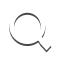-AA+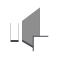A+ B+ C+= 75B+ C+ D= 62A+ B+ D= 68A+ C+ D= 65将四个“年龄和”相加可得 3( A+ B+ C+ D)= 65+ 68+ 62+ 75= 270A+ B+ C+ D= 90，故 D的年龄为 90-75= 15岁，